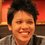# Prove that $\sqrt{536} - \sqrt{17} < 4$ without using a calculatorNote by Pi Han Goh
3 years, 11 months ago

This discussion board is a place to discuss our Daily Challenges and the math and science related to those challenges. Explanations are more than just a solution — they should explain the steps and thinking strategies that you used to obtain the solution. Comments should further the discussion of math and science.

When posting on Brilliant:

• Use the emojis to react to an explanation, whether you're congratulating a job well done , or just really confused .
• Ask specific questions about the challenge or the steps in somebody's explanation. Well-posed questions can add a lot to the discussion, but posting "I don't understand!" doesn't help anyone.
• Try to contribute something new to the discussion, whether it is an extension, generalization or other idea related to the challenge.

MarkdownAppears as
*italics* or _italics_ italics
**bold** or __bold__ bold
- bulleted- list
• bulleted
• list
1. numbered2. list
1. numbered
2. list
Note: you must add a full line of space before and after lists for them to show up correctly
paragraph 1paragraph 2

paragraph 1

paragraph 2

[example link](https://brilliant.org)example link
> This is a quote
This is a quote
    # I indented these lines
# 4 spaces, and now they show
# up as a code block.

print "hello world"
# I indented these lines
# 4 spaces, and now they show
# up as a code block.

print "hello world"
MathAppears as
Remember to wrap math in $$ ... $$ or $ ... $ to ensure proper formatting.
2 \times 3 $2 \times 3$
2^{34} $2^{34}$
a_{i-1} $a_{i-1}$
\frac{2}{3} $\frac{2}{3}$
\sqrt{2} $\sqrt{2}$
\sum_{i=1}^3 $\sum_{i=1}^3$
\sin \theta $\sin \theta$
\boxed{123} $\boxed{123}$

Sort by:

Well... \begin{aligned} 71824 &< 71825 \quad \quad \text{(take square roots)} \\ 268 &< 65 \sqrt{17} \\ 536 &< 268 + 65 \sqrt{17} \quad \quad \text{(take cube roots)} \\ \sqrt{536} &< 4+\sqrt{17} \\ \sqrt{536} - \sqrt{17} &< 4. \end{aligned} It's not enlightening but it works (and getting this without using a calculator is a little tedious, but not crazy).

The inequality at the beginning is pretty tight! The actual value is only very slightly less than 4. But anyway, note the direction of the inequality, contrary to the statement of the problem.

- 3 years, 9 months ago

Nice. I've edited the title accordingly.

Where the number of terms is small (less than 4-6), we can repeatedly square and cube things to get rid of the surds.

FYI Reversing the direction would make more sense as how someone would approach it. IE:

\begin{aligned} & \sqrt{536} - \sqrt{17} < 4 \\ \Leftrightarrow & \sqrt{536} < 4 + \sqrt{17} \\ \Leftrightarrow & 536 <268 + 65 \sqrt{17} \\ \Leftrightarrow & 268 < 65 \sqrt{17} \\ \Leftrightarrow & 71824 < 71825 \\ \end{aligned}

Staff - 3 years, 9 months ago

You have typed wrong! it should be 17 but not 18 on the fourth line of proof

- 1 year, 11 months ago

Edited, thanks.

Staff - 1 year, 11 months ago

Actually, using calculator, cuberoot(536) - sqrt(17) < 4..

- 3 years, 10 months ago

Edit: Ah yes, it is less than 4.

Staff - 3 years, 10 months ago

$4^2=16<17<25=5^2$, meaning that $4<\sqrt{17}<5$; we label this inequality as $\boxed{1}$. $8^3=216<536<729=9^3$, meaning that $8<\sqrt{536}<9$; we label this inequality as $\boxed{2}$. $\boxed{2}$-$\boxed{1}$ shows that $3 \leq \sqrt{536}-\sqrt{17} \leq 4$, meaning that $\sqrt{536}-\sqrt{17} \leq 4$

- 3 years, 9 months ago

Unfortunately, that's not how inequalities work. Do you see your mistake?

Staff - 3 years, 9 months ago